## Extract text after the last instance of a specific character

If you have a list of complex text strings that contain several delimiters (take the below screenshot as example, which contains hyphens, comma, spaces within a cell data), and now, you want to find the position of the last occurrence of the hyphen, and then extract the substring after it. This article, I will introduce some formulas for dealing with this task.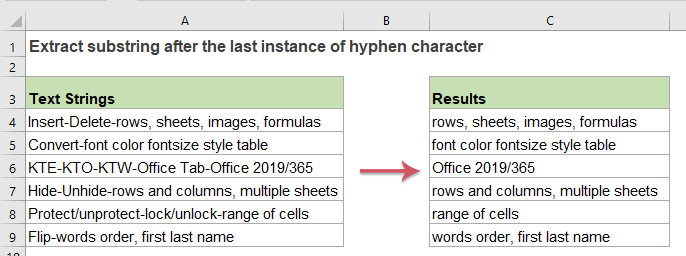#### Formula 1: Extract the substring after the last instance of a specific delimiter

In Excel, the RIGHT function which combines the LEN, SEARCH, SUBSTITUTE functions can help you to create a formula for solving this job.

1. To extract the substring after the last occurrence of the hyphen character, please enter or copy the following formula into a blank cell:

=RIGHT(A2,LEN(A2)-SEARCH("#",SUBSTITUTE(A2,"-","#",LEN(A2)-LEN(SUBSTITUTE(A2,"-","")))))

2. Then, drag the fill handle down to the cells that you want to apply this formula, and you will get the result as below screenshot shown: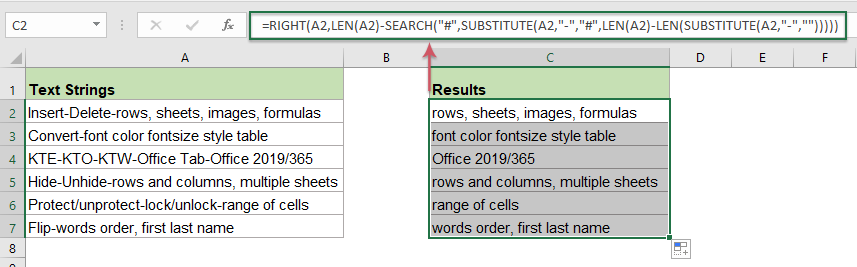##### Explanation of the formula:

1. LEN(A2)-LEN(SUBSTITUTE(A2,"-","")): This part is used to get the number of the hyphen characters in cell A2.

• LEN(A2): This LEN function returns the total number of the characters in cell A2. It will return: 44.
• SUBSTITUTE(A2,"-",""): This SUBSTITUTE function is used to replace all hyphens with nothing. And you will get the result as this: “InsertDeleterows, sheets, images, formulas”.
• LEN(SUBSTITUTE(A2,"-",""): Get the total length of the text string in cell A2 without the hyphens.
• LEN(A2)-LEN(SUBSTITUTE(A2,"-","")): Subtract the length of the text string without hyphens from the total string length to get the number of the hyphen, and this will get 2.

2. SUBSTITUTE(A2,"-","#",LEN(A2)-LEN(SUBSTITUTE(A2,"-",""))): This SUBSTITUTE function is used to replace the last occurrence of the hyphen which returned by the first part formula with a # character. And you will get this result: “Insert-Delete#rows, sheets, images, formulas”.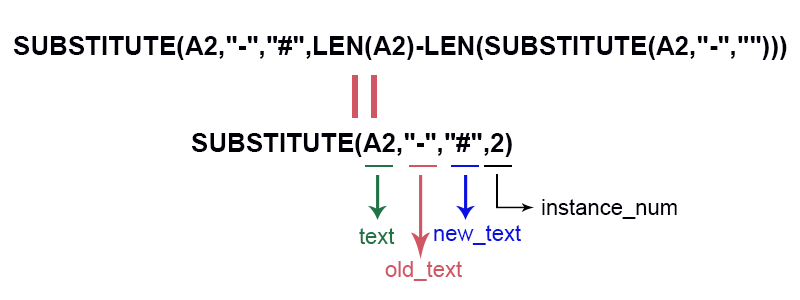3. SEARCH("#",SUBSTITUTE(A2,"-","#",LEN(A2)-LEN(SUBSTITUTE(A2,"-",""))))= SEARCH("#", "Insert-Delete#rows, sheets, images, formulas"): This SEARCH function will return the position of the # character in the text string that returned by the SUBSTUTTE function. It will get the number 14.

4. LEN(A2)-SEARCH("#",SUBSTITUTE(A2,"-","#",LEN(A2)-LEN(SUBSTITUTE(A2,"-","")))): This part of the formula will get how many characters are there after the last hyphen. This will get the number 30.

5. RIGHT(A2,LEN(A2)-SEARCH("#",SUBSTITUTE(A2,"-","#",LEN(A2)-LEN(SUBSTITUTE(A2,"-","")))))= RIGHT(A2, 30): At last, the RIGHT function is used to extract 30 characters which are returned by the formula in step 4 from the right side of the text string in cell A2.

##### Notes:

1. If you need to extract the text after the last occurrence of other delimiters, you just need to change the hyphen character with another delimiter as you need.

2. If there are no the specific delimiter in the text string, the above formula will get an error value, see screenshot: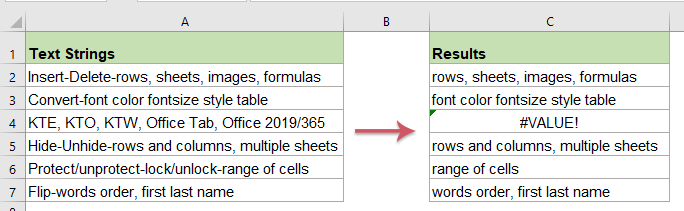To fix this error, you can enclose the above formula into the IFERROR function, please apply the following formula:

=IFERROR(RIGHT(A2,LEN(A2)-SEARCH("#",SUBSTITUTE(A2,"-","#",LEN(A2)-LEN(SUBSTITUTE(A2,"-",""))))), A2)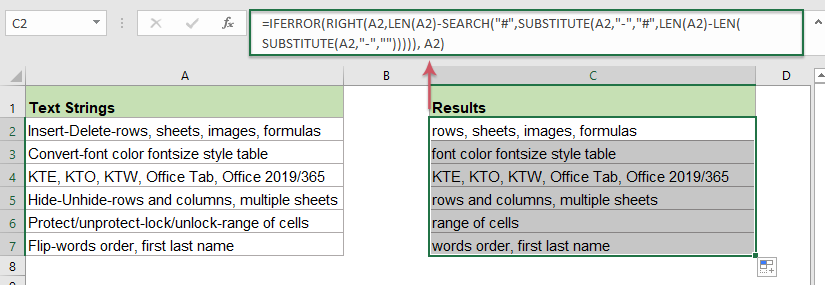#### Formula 2: Extract the substring after the last instance of a specific delimiter

Here is another simple formula which created by the TRIM, RIGHT, SUBSTITUTE, REPT and LEN functions also can help you to solve this task in Excel.

1. Please copy or enter the below formula into a blank cell where you want to get the result:

=TRIM(RIGHT(SUBSTITUTE(A2,"-",REPT(" ",LEN(A2))),LEN(A2)))

2. And then, drag the fill handle down to the cells that you want to apply this formula, and all the substrings after the last hyphens have been extracted as below screenshot shown: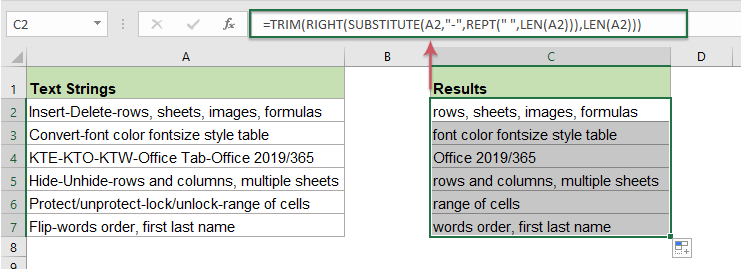##### Explanation of the formula:

1. LEN(A2): This LEN function returns the total number of the characters in cell A2. This will be recognized as the num_chars argument in RIGHT function. It will return: 44.

2. SUBSTITUTE(A2,"-",REPT(" ",LEN(A2))):

• REPT(" ",LEN(A2): This REPT function is used to get a number of space strings based on the length of cell A2.
• SUBSTITUTE(A2,"-",REPT(" ",LEN(A2))): This SUBSTITUTE function will replace the hyphen characters in cell A2 with the space strings which are returned by the REPT function.

This part formula will be recognized as the text argument in RIGHT function.

3. RIGHT(SUBSTITUTE(A2,"-",REPT(" ",LEN(A2))),LEN(A2)): This RIGHT function will get the text from the right side of text string returned by the SUBSTITUTE function.

4. TRIM(): This TRIM function is used to remove all extra spaces and only leave one space between the words.

#### Relative functions used:

• RIGHT:
• The RIGHT function extracts a specific number of characters from the right side of the text string.
• SEARCH:
• The SEARCH function can help you to find the position of a specific character or substring from the given text.
• LEN:
• The LEN function returns the number of characters in a text string.
• SUBSTITUTE:
• The Microsoft Excel SUBSTITUTE function replaces text or characters within a text string with another text or characters.
• TRIM:
• The TRIM function removes all extra spaces from text string and only keeps single spaces between words.
• REPT:
• The REPT function is used to repeat the characters a specified number of times.

#### More articles:

• Extract Multiple Lines From A Cell
• If you have a list of text strings which are separated by line breaks (that occurs by pressing Alt + Enter keys when entering the text), and now, you want to extract these lines of text into multiple cells as below screenshot shown. How could you solve it with a formula in Excel?
• Extract Nth Word From Text String In Excel
• If you have a list of text strings or sentences, now, you want to extract the specific nth word from the list as below screenshot shown. This article, I will introduce some methods for solving this job in Excel.
• Extract Text Between Parentheses From Text String
• If there is part of the text surrounded with the parentheses within the text string, now, you need to extract all the text strings between the parentheses as following screenshot shown. How could you solve this task in Excel quickly and easily?
• Extract Substring From Text String In Excel
• It may be a common task for you that you need to extract substrings from text strings, in Excel, there is not a direct function for doing this, but, with the help of the LEFT, RIGHT, MID and SEARCH functions, you can extract kinds of substrings as you need.

### The Best Office Productivity Tools

#### Kutools for Excel - Helps You To Stand Out From Crowd

 Popular Features: Find, Highlight or Identify Duplicates  |  Delete Blank Rows  |  Combine Columns or Cells without Losing Data  |  Round without Formula ... Super VLookup: Multiple Criteria  |  Multiple Value  |  Across Multi-Sheets  |  Fuzzy Lookup... Adv. Drop-down List: Easy Drop Down List  |  Dependent Drop Down List  |  Multi-select Drop Down List... Column Manager: Add a Specific Number of Columns  |  Move Columns  |  Toggle Visibility Status of Hidden Columns  |  Compare Columns to Select Same & Different Cells ... Featured Features: Grid Focus  |  Design View  |  Big Formula Bar  |  Workbook & Sheet Manager | Resource Library (Auto Text)  |  Date Picker  |  Combine Worksheets  |  Encrypt/Decrypt Cells  |  Send Emails by List  |  Super Filter  |  Special Filter (filter bold/italic/strikethrough...) ... Top 15 Toolsets:  12 Text Tools (Add Text, Remove Characters ...)  |  50+ Chart Types (Gantt Chart ...)  |  40+ Practical Formulas (Calculate age based on birthday ...)  |  19 Insertion Tools (Insert QR Code, Insert Picture from Path ...)  |  12 Conversion Tools (Numbers to Words, Currency Conversion ...)  |  7 Merge & Split Tools (Advanced Combine Rows, Split Excel Cells ...)  |  ... and more

Kutools for Excel Boasts Over 300 Features, Ensuring That What You Need is Just A Click Away...#### Office Tab - Enable Tabbed Reading and Editing in Microsoft Office (include Excel)

• One second to switch between dozens of open documents!
• Reduce hundreds of mouse clicks for you every day, say goodbye to mouse hand.
• Increases your productivity by 50% when viewing and editing multiple documents.
• Brings Efficient Tabs to Office (include Excel), Just Like Chrome, Edge and Firefox.No ratings yet. Be the first to rate!
This comment was minimized by the moderator on the site
how do you get everything after the last Nth character when there is more 'N' character that you want. Example.

1-2-3-4.ip.linodeusercontent.com.
1.2.3.4.ipv4.supernova.orange.pl.

i want to get the last bit after the IP addresses. So it ends up like this
ip.linodeusercontent.com.
pv4.supernova.orange.pl.
This comment was minimized by the moderator on the site
where "." is my Nth character
This comment was minimized by the moderator on the site
i really what to count the Nth character from the right 2 or 3 times and get everything after that, not counting the Nth character from the left because that varies. such a pain with no skills...lol
There are no comments posted here yet